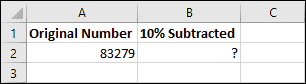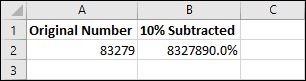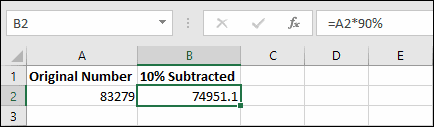# Subtracting percentages in Excel

If you’re new to Excel, it’s only a matter of time before you encounter a problem that requires the use of percentages. Using percentages in Excel formulas isn’t very intuitive for new users, which can result in a lot of confusion and frustration.

## Subtracting 10% from a number

Imagine that you have the following example workbook:You want to subtract 10% from the number in cell A2 and place the result in cell B2. To do this, you’ll need to use a very simple Excel formula.

If you’re completely new to Excel, you can learn how to create simple formulas in our completely free Basic Skills course. I’d recommend starting there if formulas are completely new to you.

Many new users would try to use the following formula:

=A2-10%

Unfortunately, this won’t have the effect that you would expect. Instead of subtracting 10% from the value of 83,279, Excel returns a percentage!So why did this happen? The reason is that Excel ‘secretly’ converts all percentages into decimal values.

## Percentages are actually decimal values

When you type 10% into Excel, Excel sees it as the value 0.1. Excel does this with all percentage values. 50% is 0.5, 75% is 0.75, and so on.

In the previous example, you were actually asking excel to subtract 0.1 from 83,279 instead of reducing the number by 10%. Excel saw your use of the % sign and tried to be helpful by formatting the result as a percentage, but in this case that just made things more confusing!

## The solution

So, knowing how Excel treats percentages, how should I subtract 10%?

One solution is to multiply the number by 90%, with one of the following formulas:

=A2*90%
=A2*0.9

Because of the way Excel handles percentages, it sees these formulas as exactly the same thing.

An alternative, but more long-winded calculation, would be to calculate 10% of the number and then subtract it from the original number, with one of these formulas:

=A2-(A2*10%)
=A2-(A2*0.1)

This will have exactly the same result and, as in the previous example, both of these formulas mean exactly the same thing to Excel.Formulas are covered in greater depth in our Essential Skills course, and our Expert Skills course expands even further, covering Excel’s more advanced and complex functions.

### 10 thoughts on “Subtracting percentages in Excel”

1.Thank you, very easy to understand.

2.thank you…you’re a great help

3.THANK U…

4.Has anyone tried this? I tried calculating and I got the wrong amount. Each formula gives something different.

1.I have tested this and the formulas all produced the same result for me. If you’re typing them exactly as shown you should get the correct results. Here are the formulas:

=A2*90%
=A2*0.9
=A2-(A2*10%)
=A2-(A2*0.1)

And here are some examples, subtracting 10% from 100:

=100*90%=90
=100*0.9=90
=100-(100*10%)=90
=100-(100*0.1)=90

5.Does not work

1.As far as I can tell all of the above examples should work correctly.

If you can explain what doesn’t work and what happens when you try it I might be able to help.

6.Yohanny cardenas

Not work for me just let #####

1.Hi Yohanny,

If you’re seeing ##### in a cell, it usually just means that the cell isn’t wide enough to display its contents. If you make the cell wider you should be able to see the result.

Scroll to Top